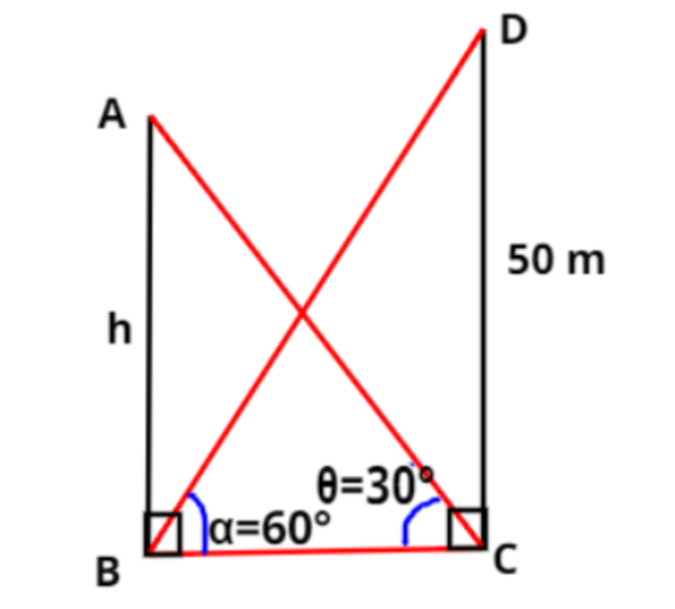Courses
Courses for Kids
Free study material
Free LIVE classes
MoreLIVE
Join Vedantu’s FREE Mastercalss

# The angle of elevation of the top of a building from the foot of the tower is ${30^ \circ }$ and the angle of elevation of the top of the tower from the foot of the building is ${60^ \circ }$. If the tower is 50 m high, find the height of the building.Verified
364.5k+ views
Hint- Here, the concept of right triangle is used along with the help of some trigonometric functions.Let us suppose a building AB of height $h$ meters and a tower CD of height 50 meters.
Given, the angle of elevation of the top of a building from the foot of the tower is $\theta = {30^ \circ }$
Also, given that the angle of elevation of the top of the tower from the foot of the building is $\alpha = {60^ \circ }$
From the figure, we can say that there are two right-angled triangles i.e., $\Delta {\text{ABC}}$ and $\Delta {\text{BCD}}$.
In right-angled $\Delta {\text{ABC}}$, we have
$\tan \theta = \dfrac{h}{{{\text{BC}}}} \Rightarrow \tan {30^ \circ } = \dfrac{h}{{{\text{BC}}}} \Rightarrow {\text{BC}} = \dfrac{h}{{\tan {{30}^ \circ }}}$
As, $\tan {30^ \circ } = \dfrac{1}{{\sqrt 3 }}$ and $\tan {60^ \circ } = \sqrt 3$
$\therefore {\text{BC}} = \dfrac{h}{{\dfrac{1}{{\sqrt 3 }}}} = h\sqrt 3$
Also, In right-angled $\Delta {\text{BCD}}$, we have
$\tan \alpha = \dfrac{{50}}{{{\text{BC}}}} \Rightarrow \tan {60^ \circ } = \dfrac{{50}}{{h\sqrt 3 }} \Rightarrow \sqrt 3 = \dfrac{{50}}{{h\sqrt 3 }} \Rightarrow h = \dfrac{{50}}{{{{\left( {\sqrt 3 } \right)}^2}}} = \dfrac{{50}}{3}$
Therefore, the height of the building is $\dfrac{{50}}{3}$ meters.

Note- In any right angle triangle, the hypotenuse is the side opposite to right angle, the perpendicular is the side opposite to the considered acute angle and base is the left side. Also, trigonometric function $\tan \theta$ is the ratio of the perpendicular to the hypotenuse in the right triangle.

Last updated date: 22nd Sep 2023
Total views: 364.5k
Views today: 9.64k